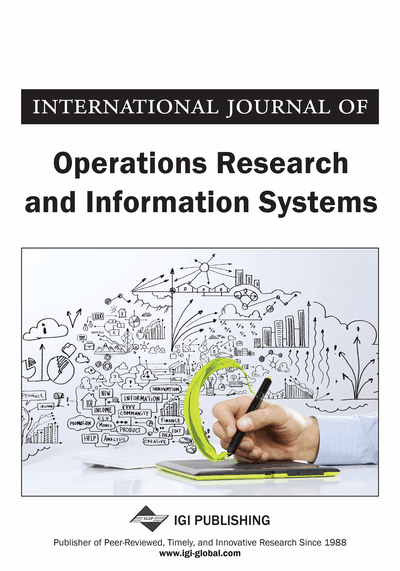# Multiobjective Transportation Problem Using Fuzzy Decision Variable Through Multi-Choice Programming

Gurupada Maity (Vidyasagar University, Department of Applied Mathematics with Oceanology and Computer Programming, Midnapore, India) and Sankar Kumar Roy (Vidyasagar University, Department of Applied Mathematics with Oceanology and Computer Programming, Midnapore, India)
DOI: 10.4018/IJORIS.2017070105

## Abstract

This paper analyzes the study of Multiobjective Transportation Problem (MOTP) under the consideration of fuzzy decision variable. Usually, the decision variable in a Transportation Problem is taken as real variable. But, in this paper, the decision variable in each node is selected from a set of multi-choice fuzzy numbers. Inclusion of multiple objectives into transportation problem with fuzzy decision variable makes it a Multiobjective Fuzzy Transportation Problem (MOFTP). In this paper, a new formulation of mathematical model of MOFTP with fuzzy goal of each objective function is enlisted. Thereafter the solution technique of the formulated model is described through multi-choice goal programming approach. Finally, a numerical example is presented to show the feasibility and usefulness of this article.
Article Preview
Top

## Introduction

Transportation problem acts an important role for decision-making problem to cover the real-life situations. The transportation problem can be delineated as a special case of a linear programming problem and its model is used to determine an optimal solution of the decision-making problem. The classical transportation problem determines how many units of a commodity are to be shipped from each point of origin to various destinations, satisfying source availabilities and destination demands, while minimizing the total cost of transportation along with cutting down the costs per unit of items for the purchasers.

The basic transportation problem was originally developed by Hitchcock (1941) and later developed independently by Koopmans (1949). Transportation problem with single objective function is not enough to tackle various real-life decision-making problems due to our present competitive market scenario. So, we have to introduce multiobjective transportation problem to accommodate such real-life situations. A good number of works in this ground has been done by several researchers like Verma et al. (1997), Waiel (2001), Mahapatra et al. (2010), Datta et al. (2010), Roy and Mahapatra (2011), Das et al. (2011), Mahapatra et al. (2013), Midya and Roy (2014), Beauchamp et al. (2015), Maity and Roy (2016) and many others.

Initially, fuzzy set theory has been considered as a tool to solve multiobjective optimization problems (Zimmerman 1978). Furthermore, the notion of fuzzy numbers is introduced in the transportation parameters (cost, supply and demand) of a transportation problem to make it a fuzzy transportation problem. Kumar and Kaur (2011) developed a methodology for solving fuzzy transportation problems based on classical transportation methods. Ebrahimnejad et al. (2011) proposed an algorithm for bounded linear programming with fuzzy cost coefficients in a decision-making problem. Marbini et al. (2011) introduced an interactive approach on data envelopment analysis with fuzzy parameters. A study on multi-criteria futuristic fuzzy decision hierarchy and its application in a tourism industry has been presented by Singh et al. (2015). Pattnaik (2015) incorporated a study on decision-making approach to fuzzy linear programming (FLP) problems with post optimal analysis. Kumar and Hussain (2016) presented a simple methodology for solving a real-life assignment problem under intuitionistic fuzzy environment. To the best of our knowledge, no one has been introduced the concept of decision variable in transportation problem as fuzzy variable. Here, we assume that the expectations in the destinations of transportation problem are fuzzy numbers and they are treated as fuzzy goals. In the destinations, there are multi-choice fuzzy expectations. In this situation, decision maker would have to take a decision of supplying goods in such a way that the profit would maximize, keeping the best possible fulfillment of requirement at the destinations. Again, it is not necessarily true that in a transportation problem at each node there is an allocation, it depends on the best fit of the problem. In this case, when there is no need of allocation in a cell, then we assign a crisp goal “0” with high priority value. In our proposed transportation problem, the requirement in the allocated cells are one among a multi-choice fuzzy numbers along with “0”. With this assumption, we design a transportation problem whose decision variables are fuzzy. This situation of decision-making problem is solved using the multi-choice goal programming approach.

## Complete Article List

Search this Journal:
Reset
Open Access Articles: Forthcoming
Volume 11: 4 Issues (2020): 1 Released, 3 Forthcoming
Volume 10: 4 Issues (2019)
Volume 9: 4 Issues (2018)
Volume 8: 4 Issues (2017)
Volume 7: 4 Issues (2016)
Volume 6: 4 Issues (2015)
Volume 5: 4 Issues (2014)
Volume 4: 4 Issues (2013)
Volume 3: 4 Issues (2012)
Volume 2: 4 Issues (2011)
Volume 1: 4 Issues (2010)
View Complete Journal Contents Listing